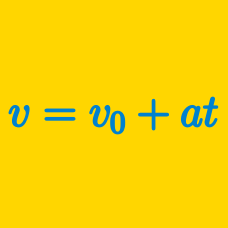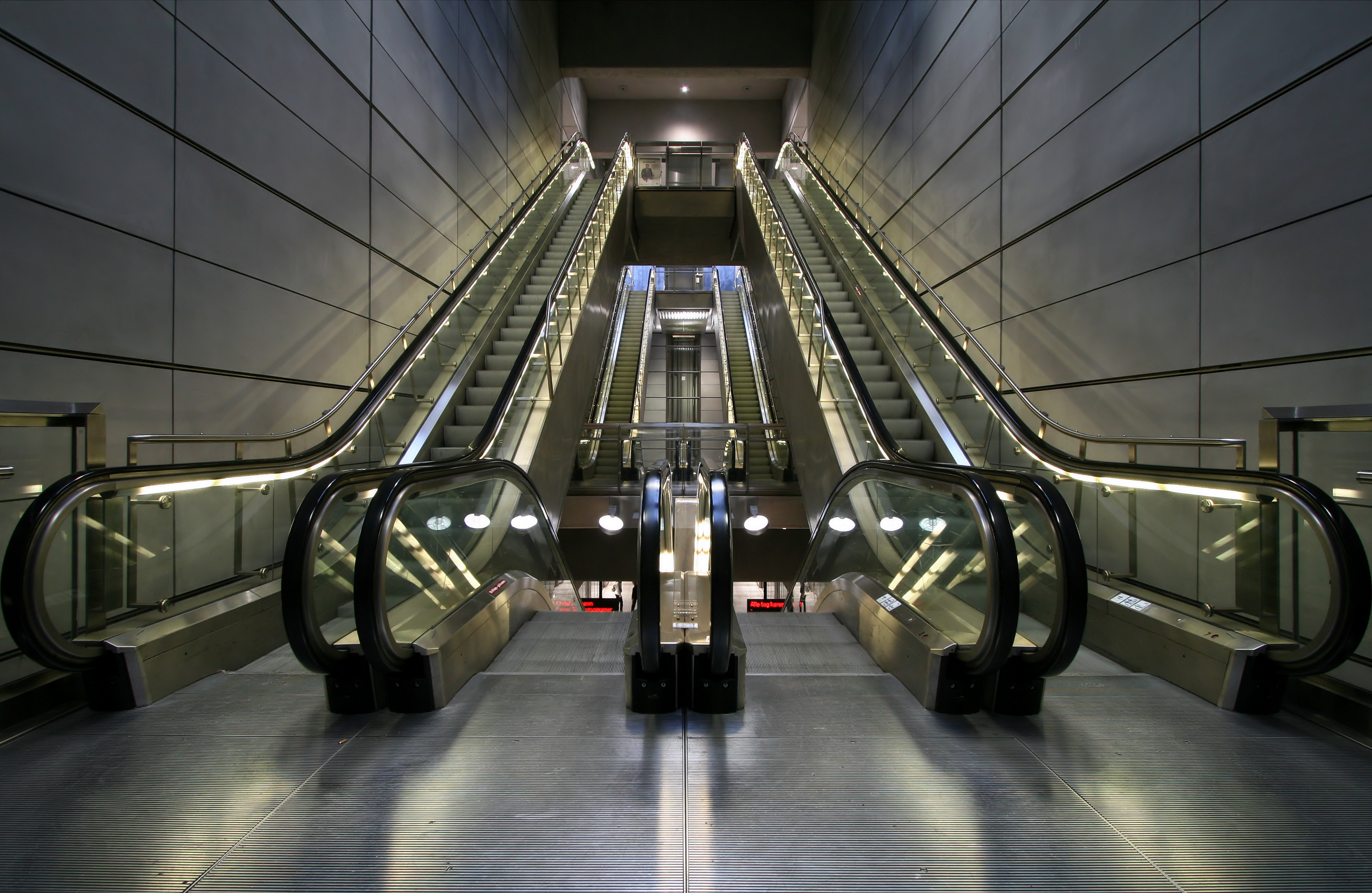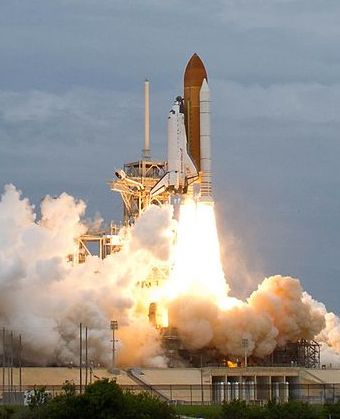Classical Mechanics

# Problem solving with velocity and acceleration - BasicIn a subway station, there are two escalators going upwards toward the exit. One is broken while the other one is working. If a man walks up the broken escalator, it takes $$54$$ seconds for him to leave the station. If he takes the working escalator while standing still, it takes $$28$$ seconds for him to leave the station. If this man walks while taking the working escalator, approximately how long will it take for him to leave the station?

Assume that the escalator and man are both move with constant respective velocities.

The length of a clock's second-hand is $$45\text{ mm}.$$ What is the average velocity of the end point of this second hand during any thirty seconds of time?

An object is positioned at $$x=0$$ at time $$t=0.$$ It accelerates at a rate of $$2.3\text{ m/s}^2$$ for $$2.6$$ seconds, then it accelerates at a rate of $$-5.2\text{ m/s}^2$$ for $$2.4$$ seconds. What is the approximate velocity of the object at $$t=4.4$$ seconds?Two spaceships initially at rest take off to travel a distance of $$2000\text{ km}.$$ One spaceship accelerates uniformly at a rate of $$40 \text{ km/s}^2$$, and the second one moves with a constant acceleration of $$10 \text{ km/s}^2.$$ How many seconds earlier will the first spaceship reach its destination?

The position of an object moving along the $$x$$-axis with constant acceleration is described as $x=\frac{7}{2}t^2+3t+6.$ If $$t$$ is in seconds and $$x$$ is in meters, what is the acceleration of this object?

×

Problem Loading...

Note Loading...

Set Loading...